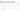# How does result mapping work?

##### What is outcome mapping?

When a user is taking a quiz, each answer they give should move them closer to receiving an outcome. That outcome could be a categorization like "you're a dog person", it could be a ranking like "you're an expert", or it could simply be a number of questions they answered correctly.

For quizpipe to know which answer relates to which outcome, or if an answer is right or wrong, each answer needs to be "mapped".

##### Category Outcome Type MappingOnce you have your categories created, you can move on to creating your questions and answers by selecting the Next button at the bottom of the pageWhen you've found your way to the questions page, write up a question and a few answers. For the purposes of this article we won't discuss how you set up questions and answers. If you're struggling to get this set up, please read "How to set u questions and answers".

Once your answers are created, either tick the checkbox to the side of the questionor pick the Mapping tab on the left sidebar menu.Once you've opened this menu you will see each of your answers with a drop down that allows you to pick an outcome.

You have to pick at least one answer to map an outcome for on each question.

When the user is taking your quiz, each answer they pick that is mapped to an outcome will earn them one point towards that outcome. For example, if they pick three answers that are mapped to "You're a dog" then they will earn three points towards that outcome. At the end of the quiz, whichever outcome has the most points will be displayed on the results page.

##### Number and Percentage Outcome Type MappingNumber and percentage outcomes tell the user how many answers they picked correctly at the end of the quiz. For that reason, when you're mapping the outcomes you only need to nominate which answer(s) are correct.

For each question you need to pick at least one answer that is correct.

To do this, click on the checkbox next to the answeror pick the Mapping tab on the left sidebar menu.Once you've opened this menu you will see each of your answers with a checkbox that allows you to mark the answer as correct.

You can mark multiple answers as correct. You must pick at least one answer as correct for each question.

As the user takes the quiz, each answer they pick correctly will be added to their score total which will display on the quiz results page.

##### Ranking Outcome Type MappingRanking outcomes work similarly to the number and percentage outcome type but rather than explicitly telling the user how many answers they got right at the end of the quiz, they are "ranked" into a category.

Before you start to map your answers, you'll need to define what range of results equates to each rank. For example:
0 - 19 = Poor
20 - 39 = Below average
40 - 59 = Average
60 - 79 = Good
80 - 100 = Excellent

Each correct answer the user selects will be worth a number of points. The number is points is equal to:

`(100 points / number of correct answers)`

For example:

A quiz has 20 questions and only one correct answer for each question. The number of points each correct answer is worth is:

`(100 points / 20 possible correct answers) = 5 points`

In this case, if a user answered 10 correct questions (50 points) they would be given the outcome of Average, since any score between 40 and 59 is qualified as Average.

To map the correct answers for a ranking quiz outcome type, click click on the checkbox next to any answeror pick the Mapping tab on the left sidebar menu.Once you've opened this menu you will see each of your answers with a checkbox that allows you to mark the answer as correct.

You can mark multiple answers as correct. You must pick at least one answer as correct for each question.